Courses
Courses for Kids
Free study material
Free LIVE classes
More

# Skip Counting by 10## Introduction to Skip Counting

Skip counting is a method of counting a particular number by adding again and again every time to the previous number. This can be forwarded by a number which is greater than 1.

For example, skip counting of 4 then we get as a resultant 4, 8, 12, 16... and so on.

There are two types of skip counting

• Forward Skip Counting

• Backward Skip Counting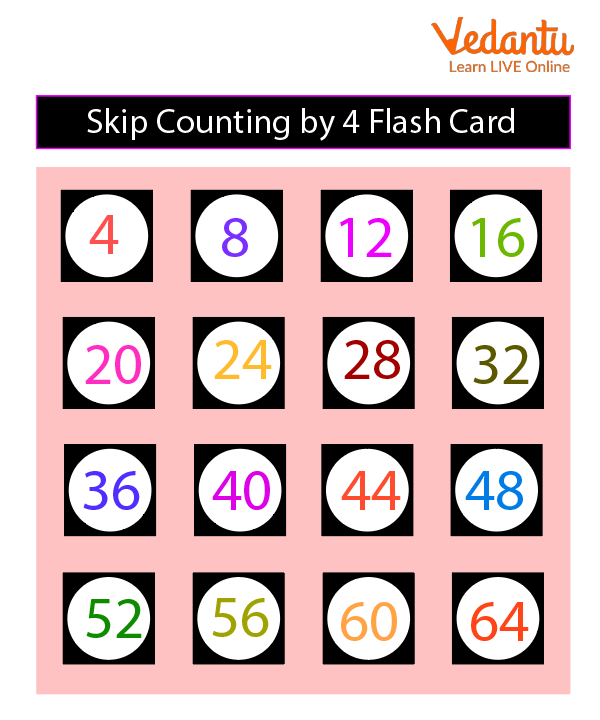Flash Example

Skip counting is a quick way to count equal groups and a different strategy to introduce learning tables. Skip count is also an important part to get new science about numbers because it helps a student to see different patterns with numbers and learn with fun.

## Skip Counting By 10

Skip counting is a process to count a particular number by adding every time to the respective previous number. When we add 10 or tense to again and again to the previous number gives us a particular table of 10. In other words we count by tens the rate that we are adding a number is by a unit of 10. Skip counting by 10 can also mean by adding or subtracting 10 each and every time to the previous number.

The basic concept of escape counting by tens (10) is a unique skill to learn to jump a respective number from counting to the basic addition method. Skip count by 10 is very easy and quick to learn about numbers.

## How to Count by 10:

As we know the concept of skip count by 10 is a very quick and easy method. We can count by 10 to the respective types:

Forward Skip Counting by 10: when we use forward skip count, we add numbers to forward direct to a number. We have to follow respective pattern for this method,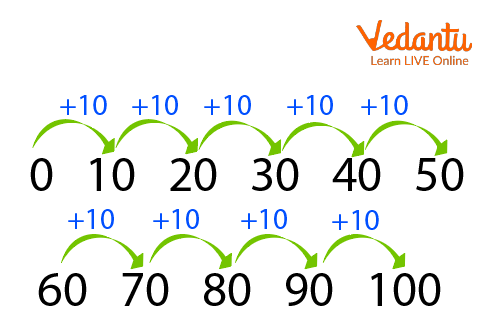Skip Forwarding by 10.

0+10 = 10

10+10 = 20

20+10 = 30

Backward Skip Counting by 10: when we perform backward skip count we have to subtract 10 from particular numbers,

for example –

100 – 10 = 90

90 – 10 = 80

## 2 Tens are 20:

In the term of skip counting by 10, tens are adding on 10, the respective answer was 20. In other words, 2 tens are 20, representing that if we are adding 10 to again with 10, we get 20 as a skip account number by 10. We can also say that two times out of 10 is represented as 20.

• 10 + 10 = 20

• 2 * 10 =20

## Counting by 10s Chart Printable:

This chat will help us to get information about the concept of skip counting by the number 10.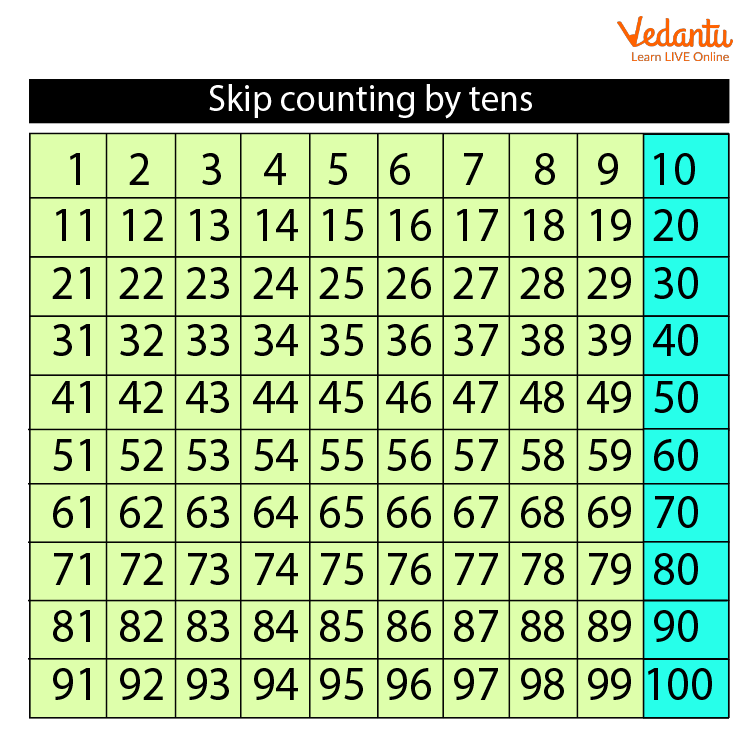Skip Counting By 10.

## Skip Counting By 10 to 100 Worksheet:

This worksheet will help the students to understand the concept of skip counting by 10 to 100.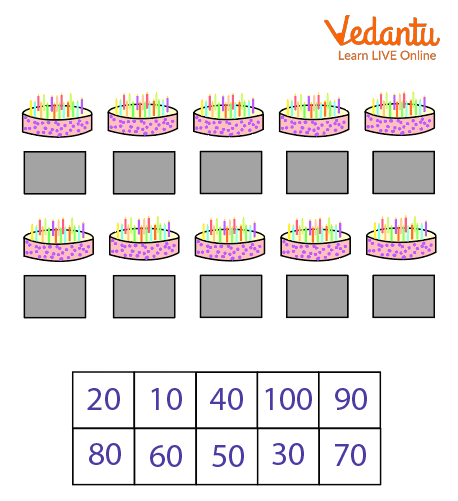Question of Skip Counting

Ans: 10, 20, 30, 40, 50, 60, 70, 80, 90, 100.

## Solved Example

1. Complete the following.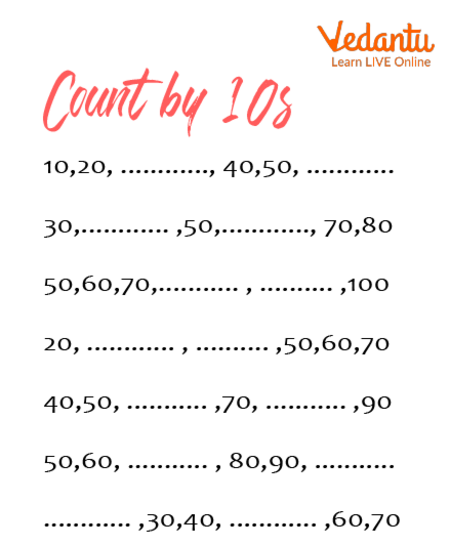Count By 10

Ans: 30,60

40,60

80,90

30,40

60,80

20,50

## Solved Questions

1. Count by 10 and fill in the missing numbers.

 10 30 50 70 100 130

Ans: 20, 40, 60, 80, 90 110, 120, 140, 150

2. Count objects in tens and write the numbers in the boxes.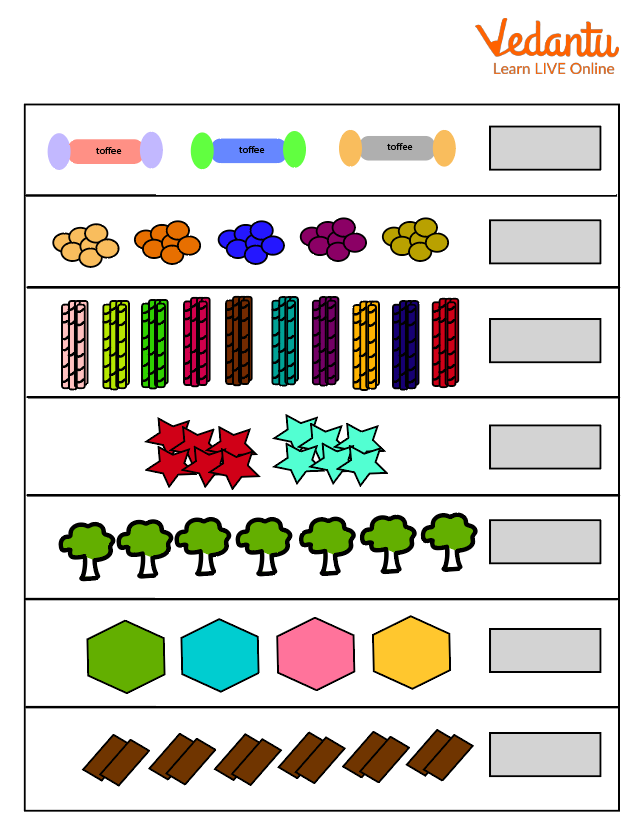Questions

Ans: 30, 50, 100, 20, 70, 40, 60.

## Summary

Skip counting helps every student to count many things very easily and quickly. It also helps to learn multiplication terms as a form of tables. The skip counting helps students to count and learn new things about the number system. This concept also helps the student for basic tasks such as getting information about time or getting knowledge about how to deal with money.

Last updated date: 29th Sep 2023
Total views: 82.5k
Views today: 0.82k

## FAQs on Skip Counting by 10

1.What are the benefits of  skip counting?

Learning about the mathematical purpose of multiplication can be facilitated by the use of skip counting. Students may learn the tables more quickly.

2. Give any one example of skip counting that we can use in our daily life?

Skip counting is an important mathematical ability that will probably make multiplication easier for kids. This technique can be used by students to count objects or money of a specific denomination.

3. Where do we use skip counting in our daily life?

Use of skip counting –

1. Telling time

2. Counting money